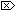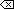The Open Group Base Specifications Issue 6
IEEE Std 1003.1-2001

#### NAME

erfc, erfcf, erfcl - complementary error functions

#### SYNOPSIS

```#include <math.h> double erfc(double x); float erfcf(float x); long double erfcl(long double x); ```

#### DESCRIPTION

[CX]The functionality described on this reference page is aligned with the ISO C standard. Any conflict between the requirements described here and the ISO C standard is unintentional. This volume of IEEE Std 1003.1-2001 defers to the ISO C standard.These functions shall compute the complementary error function 1.0 - erf(x).

An application wishing to check for error situations should set errno to zero and call feclearexcept(FE_ALL_EXCEPT) before calling these functions. On return, if errno is non-zero or fetestexcept(FE_INVALID | FE_DIVBYZERO | FE_OVERFLOW | FE_UNDERFLOW) is non-zero, an error has occurred.

#### RETURN VALUE

Upon successful completion, these functions shall return the value of the complementary error function.

If the correct value would cause underflow and is not representable, a range error may occur and [MX]either 0.0 (if representable), oran implementation-defined value shall be returned.

[MX]If x is NaN, a NaN shall be returned.

If x is ±0, +1 shall be returned.

If x is -Inf, +2 shall be returned.

If x is +Inf, +0 shall be returned.

If the correct value would cause underflow and is representable, a range error may occur and the correct value shall be returned.#### ERRORS

These functions may fail if:

Range Error
The result underflows.

If the integer expression (math_errhandling & MATH_ERRNO) is non-zero, then errno shall be set to [ERANGE]. If the integer expression (math_errhandling & MATH_ERREXCEPT) is non-zero, then the underflow floating-point exception shall be raised.

The following sections are informative.

None.

#### APPLICATION USAGE

The erfc() function is provided because of the extreme loss of relative accuracy if erf(x) is called for large x and the result subtracted from 1.0.

Note for IEEE Std 754-1985 double, 26.55 < x implies erfc( x) has underflowed.

On error, the expressions (math_errhandling & MATH_ERRNO) and (math_errhandling & MATH_ERREXCEPT) are independent of each other, but at least one of them must be non-zero.

None.

#### FUTURE DIRECTIONS

None.

erf() , feclearexcept() , fetestexcept() , isnan() , the Base Definitions volume of IEEE Std 1003.1-2001, Section 4.18, Treatment of Error Conditions for Mathematical Functions, <math.h>

#### CHANGE HISTORY

First released in Issue 1. Derived from Issue 1 of the SVID.

#### Issue 5

The DESCRIPTION is updated to indicate how an application should check for an error. This text was previously published in the APPLICATION USAGE section.

#### Issue 6

The erfc() function is no longer marked as an extension.

These functions are split out from the erf() reference page.

The DESCRIPTION, RETURN VALUE, ERRORS, and APPLICATION USAGE sections are revised to align with the ISO/IEC 9899:1999 standard.

IEC 60559:1989 standard floating-point extensions over the ISO/IEC 9899:1999 standard are marked.

End of informative text.

UNIX ® is a registered Trademark of The Open Group.
POSIX ® is a registered Trademark of The IEEE.
[ Main Index | XBD | XCU | XSH | XRAT ]